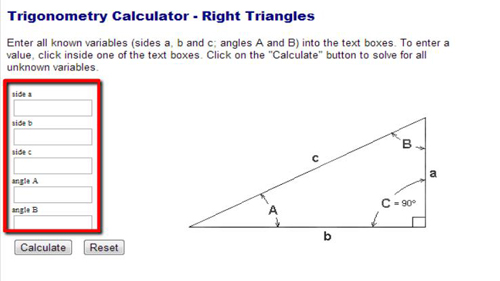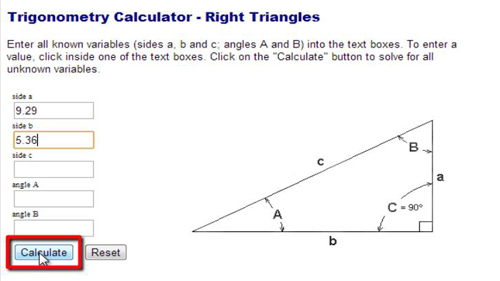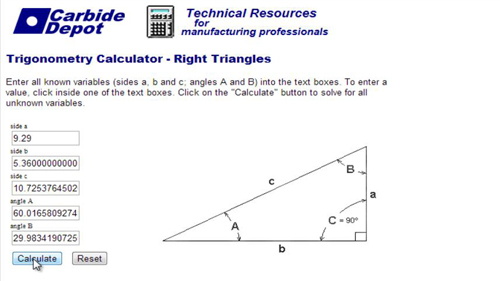## How to Use Calculator for Trigonometry

This tutorial will demonstrate how to use an Online Calculator for Trigonometry.

Step # 1 – Access the Online Trig Calculator

Go to Carbidedepot site. On the right side of the page, you will see a right angled triangle, and on the left side, you will see a form where you can input numbers.Step # 2 –Entering the Known Variables

The form on the left will be divided into five sections, which are sides a, b and c, as well as angles A and B. It is not necessary to enter data into each section; you can simply enter the variables you have and the calculator will do the rest. For this tutorial, enter “9.29” under “side a” and “5.36” under “side b.” After doing this, click the “Calculate” button.Step # 3 –Computing the Unknown Variables

The calculator for trigonometry will now compute the answer, automatically filling out the other sections. We provided the sides for a and b, and it has now given us “10.72” for side c, “60.01” for angle A, and “29.98” for angle B.